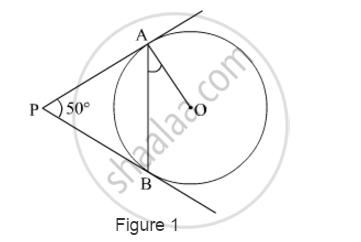# In Fig. 1, PA and PB are tangents to the circle with centre O such that ∠APB = 50°. Write the measure of ∠OAB. - Mathematics

In Fig. 1, PA and PB are tangents to the circle with centre O such that ∠APB = 50°. Write the measure of ∠OAB.#### Solution

PA and PB are tangents drawn from an external point P to the circle.

∴ PA = PB      (Length of tangents drawn from an external point to the circle are equal.)

In ∆PAB,

PA = PB

PBA = PAB     .....(1)     (Angles opposite to equal sides are equal.)

Now,

APB + PBA + PAB = 180°

⇒ 50º + PAB + PAB = 180°    [Using (1)]

⇒ 2PAB = 130°

PAB =130^@/2= 65°

We know that radius is perpendicular to the tangent at the point of contact.

OAP = 90°         (OA ⊥ PA)

PAB + OAB = 90°

⇒ 65° + OAB = 90°

OAB = 90° − 65° = 25°

Hence, the measure of OAB is 25°.

Concept: Concept of Circle - Centre, Radius, Diameter, Arc, Sector, Chord, Segment, Semicircle, Circumference, Interior and Exterior, Concentric Circles
Is there an error in this question or solution?
2014-2015 (March) Delhi Set 1
Share